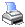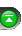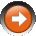﻿ PlotColors
 NinjaScript > Language Reference > Indicator > PlotColorsDefinition

Holds an array of color series objects holding historical bar colors. A color series object is added to this array when calling the Add() method in a Custom Indicator for plots. Its purpose is to provide access to the color property of all bars.

Property Value

An array of color series objects.

Syntax
PlotColors[int PlotIndex][int barsAgo]

Examples

 protected override void Initialize() {     // Add two plots     Add(new Plot(Color.Blue, PlotStyle.Line, "Upper"));     Add(new Plot(Color.Orange, PlotStyle.Line, "Lower")); }   protected override void OnBarUpdate() {     // Sets values to our two plots     Upper.Set(SMA(High, 20));     Lower.Set(SMA(Low, 20));       // Color the Upper plot based on plot value conditions     if (Rising(Upper))          PlotColors = Color.Blue;     else if (Falling(Upper))          PlotColors = Color.Red;     else          PlotColors = Color.Yellow;       // Color the Lower plot based on plot value conditions     if (Rising(Lower))          PlotColors = Color.Blue;     else if (Falling(Lower))          PlotColors = Color.Red;     else          PlotColors = Color.Yellow; }